# What Is An Arithmetic Sequence? (8 Common Questions Answered)

Arithmetic sequences are used in mathematics whenever we have a sequence of numbers that increases in a predictable way at each step.  However, there are a few things you should know about these sequences.

So, what is an arithmetic sequence?  An arithmetic sequence is a sequence of numbers that increases by a constant amount at each step. The difference between consecutive terms in an arithmetic sequence is always the same. The difference d is called the common difference, and the nth term of an arithmetic sequence is an = a1 + d(n – 1).

Of course, an arithmetic sequence can have positive, negative, or zero terms.  The common difference can also be positive, negative, or zero.

Let’s get started.

## What Is An Arithmetic Sequence?

An arithmetic sequence (or arithmetic progression) is a sequence of numbers that increases by the same constant amount at each step.  That is, the difference between two consecutive terms in an arithmetic sequence is always the same.

This difference, d, is called the common difference of the arithmetic sequence.  If the nth term of an arithmetic sequence is an, then the common difference d is:

• d = an+1 – an

Note that this formula also tells us how to find the next term in the sequence from the previous term.  If we solve for an+1, we get:

• an+1 = an + d

We can use any positive value of n to find the value of d.  For example, if we choose n = 1, then we only need the first two terms of the arithmetic sequence to find d:

• d = a2 – a1

If we choose n = 10, we would need the 10th and 11th terms of the arithmetic sequence to find d:

• d = a11 – a10

### How To Find The Common Difference In An Arithmetic Sequence

If we have two consecutive terms in an arithmetic sequence, we can simply take their difference to find the common difference d (as we did in the examples above).  Just remember to subtract in the correct order, since subtraction is not commutative.

For example, given a1 = 4 and a2 = 6, we would calculate:

• d = a2 – a1
• =6 – 4
• =2

The common difference for this arithmetic sequence would be 2.  It would be incorrect to calculate 4 – 6 = -2.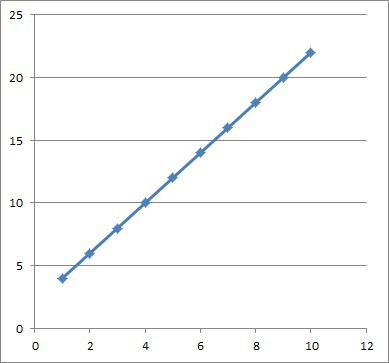The first several numbers in the arithmetic sequence with first term 4 and common difference 2. Note that the slope of the resulting line is positive (+2, the same as the common difference).

In general, the term with the larger index (the number in the subscript) comes first, and the term with the smaller index is subtracted from it.

If we have two terms that are not consecutive, we need to subtract them (again, in the proper order) and then divide by the difference of the index.

So, given two terms am and a in an arithmetic sequence (with m < n), we would find the common difference d with the formula:

• d = (an – am) / (n – m)

For example, given the two terms a3 = 7 and a12 = 34 in an arithmetic sequence, we would use the formula above to calculate d with m = 3, n = 12, am = 7, and an = 34:

• d = (an – am) / (n – m)
• d = (a12 – a3) / (12 – 3)
• d = (34 – 7) / (9)
• d = 27 / 9
• d = 3

So, the common difference for this arithmetic sequence is d = 3.  With this information, we can work backwards to find the first term a1 if we want to:

• a3 = 7
• a2 = 4  [since a3 = a2 + d, with a3 = 7 and d = 3]
• a1 = 1  [since a2 = a1 + d, with a2 = 4 and d = 3]

We can find a general formula for the first term a1 by solving the equation from before with m = 1:

• d = (an – am) / (n – m)
• d = (an – a1) / (n – 1)  [substitute m = 1]
• d(n – 1) = an – a1  [multiply both sides by d]
• d(n – 1) – an = -a1  [subtract an from both sides]
• an – d(n – 1) = a1  [multiply by -1 on both sides]

So, given the nth term an and the common difference d of an arithmetic sequence, we can find the first term a1 by the equation a1 = an – d(n – 1).

### How To Find The Nth Term Of An Arithmetic Sequence

To find a general formula for the nth term of an arithmetic sequence, we need the first term a1 and the common difference d.

We can solve the same equation as before, but for an instead of a1:

• d = (an – am) / (n – m)
• d = (an – a1) / (n – 1)  [substitute m = 1]
• d(n – 1) = an – a1  [multiply both sides by d]
• d(n – 1) + a1 = an  [add a1 to both sides]

So, given the 1st term a1 and the common difference d of an arithmetic sequence, we can find the nth term an by the equation an = a1 + d(n – 1).

#### Example: How To Find The Nth Term Of An Arithmetic Sequence

Let’s say we are given the terms a5 = 12 and a8 = 21 of an arithmetic sequence.  First, we will find d by the equation from earlier:

• d = (an – am) / (n – m)
• d = (a8 – a5) / (8 – 5)  [use m = 5, n = 8]
• d = (21 – 12) / (3)
• d = 9 / 3
• d = 3

Now we need to find a1.  We will use the formula for a1 that we found earlier, along with a5 = 12:

• a1 = an – d(n – 1)
• a1 = a5 – 3(5 – 1)  [n = 5, d = 3]
• a1 = 12 – 3(4)  [a5 = 12]
• a1 = 12 – 12
• a1 = 0

Now we can find the formula for the nth term an from the equation from earlier:

• an = a1 + d(n – 1)
• an = 0 + 3(n – 1)  [a1 = 0, d = 3]
• an = 3n – 3

We can check this formula for the 3 terms we already have to confirm:

• a1 = 3(1) – 3 = 3 – 3 = 0 (correct!)
• a5 = 3(5) – 3 = 15 – 3 = 12 (correct!)
• a8 = 3(8) – 3 = 24 – 3 = 21 (correct!)

So, the formula for the nth term of this arithmetic sequence is an = 3n – 3.

The first several terms of the arithmetic sequence are 0, 3, 6, 9, 12, 15, 18, 21, 24, …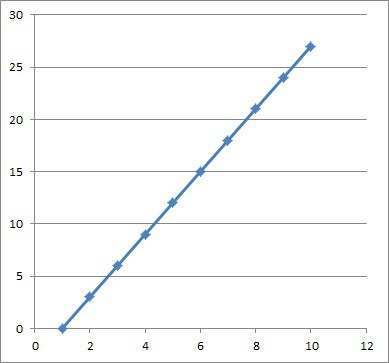The first several numbers in the arithmetic sequence with first term 0 and common difference 3. Note that the slope of the resulting line is positive (+3, the same as the common difference).

### What Is The Recursive Formula For An Arithmetic Sequence?

The recursive formula for an arithmetic sequence is the one involving the next term, the previous term, and the common difference d (the same one we saw earlier):

• an+1 = an + d

It is called recursive because it refers to a previous term to find the next term.

### Can Arithmetic Sequences Be Negative?

Arithmetic sequences can have negative terms.  In fact, an arithmetic sequence will have all of its terms negative if:

• the first term a1 is negative
• the common difference d is negative or zero (nonpositive)

Of course, an arithmetic sequence may have some terms, one term, or no terms negative.

#### Example of An Arithmetic Sequence That Is Negative (All Terms Negative)

Consider the arithmetic sequence with first term a1 = -5 and common difference d = -4.   All of the terms in this sequence will be negative, since:

• the first term a1 is negative
• the common difference d is negative or zero (nonpositive)

We can prove this with the general formula for the nth term of an arithmetic sequence:

• an = a1 + d(n – 1)  [formula for nth term of any arithmetic sequence]
• an = -5 + -4(n – 1)  [a1 = -5, d = -4]
• an = -5 – 4n + 4
• an = -4n – 1
• an = -(4n + 1)

Since 4n + 1 is positive for any natural number n, we know that an = -(4n + 1) will be negative for any natural number n.  Thus, every term of this arithmetic sequence will be negative.

The terms will be -5, -9, -13, -17, -21, -25, -29, -33, -37 …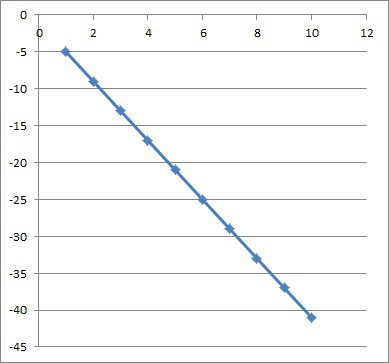The first several numbers in the arithmetic sequence with first term -5 and common difference -4. Note that the slope of the resulting line is negative (-4, the same as the common difference).

### Can Arithmetic Sequences Have Decimals Or Fractions?

Arithmetic sequences can have decimals or fractions.  This can happen if either:

• the first term a1 involves decimals or fractions (a1 is not a whole number)
• the common difference d involves decimals or fractions (d is not a whole number)

#### Example of An Arithmetic Sequence With Decimals

Consider the arithmetic sequence with first term a1 = 3.4 and common difference d = 2.   All of the terms in this sequence will have decimals, since:

• the first term a1 is not a whole number
• the common difference d is a whole number

The terms will be 3.4, 5.4, 7.4, 9.4, 11.4, 13.4, 15.4, 17.4, 19.4, …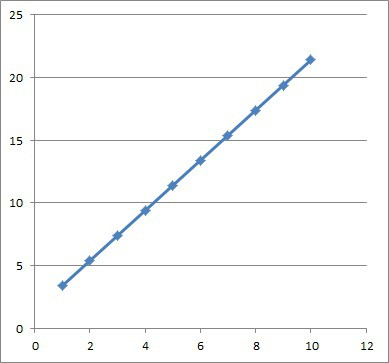The first several numbers in the arithmetic sequence with first term 3.4 and common difference 2. Note that the slope of the resulting line is positive (+2, the same as the common difference).

### Do Arithmetic Sequences Converge?

Arithmetic sequences do not converge unless the common difference d is zero (in that case, all of the terms are the same value, and the sequence converges to that value).

We can see that an arithmetic sequence with nonzero d does not converge by looking at the formula for the nth term:

• an = a1 + d(n – 1)

As n gets larger (grows without bound), the term d(n – 1) will also get larger in absolute value.  This means that there is no boundary on the value of an: at each step, it grows by d, never settling at one value.

### Is The Fibonacci Sequence An Arithmetic Sequence?

The Fibonacci Sequence is not an arithmetic sequence, since it does not have the proper form: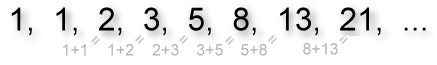Each term in the Fibonacci sequence is defined as the sum of the previous two terms (the first two terms are both taken to be one).

To find the nth term of the Fibonacci sequence, we use the formula:

• an = an – 1 + an – 2

where a1 = 1 and a2 = 1.

Remember the key difference:

• Arithmetic Sequence: to get the next term, take the previous term and add a constant value (the common difference d, which never changes)
• Fibonacci Sequence: to get the next term, take the previous term and add the 2nd previous term (the terms vary, so it is not a constant value being added).

The first several terms of the Fibonacci sequence are 1, 1, 2, 3, 5, 8, 13, 21, 34, …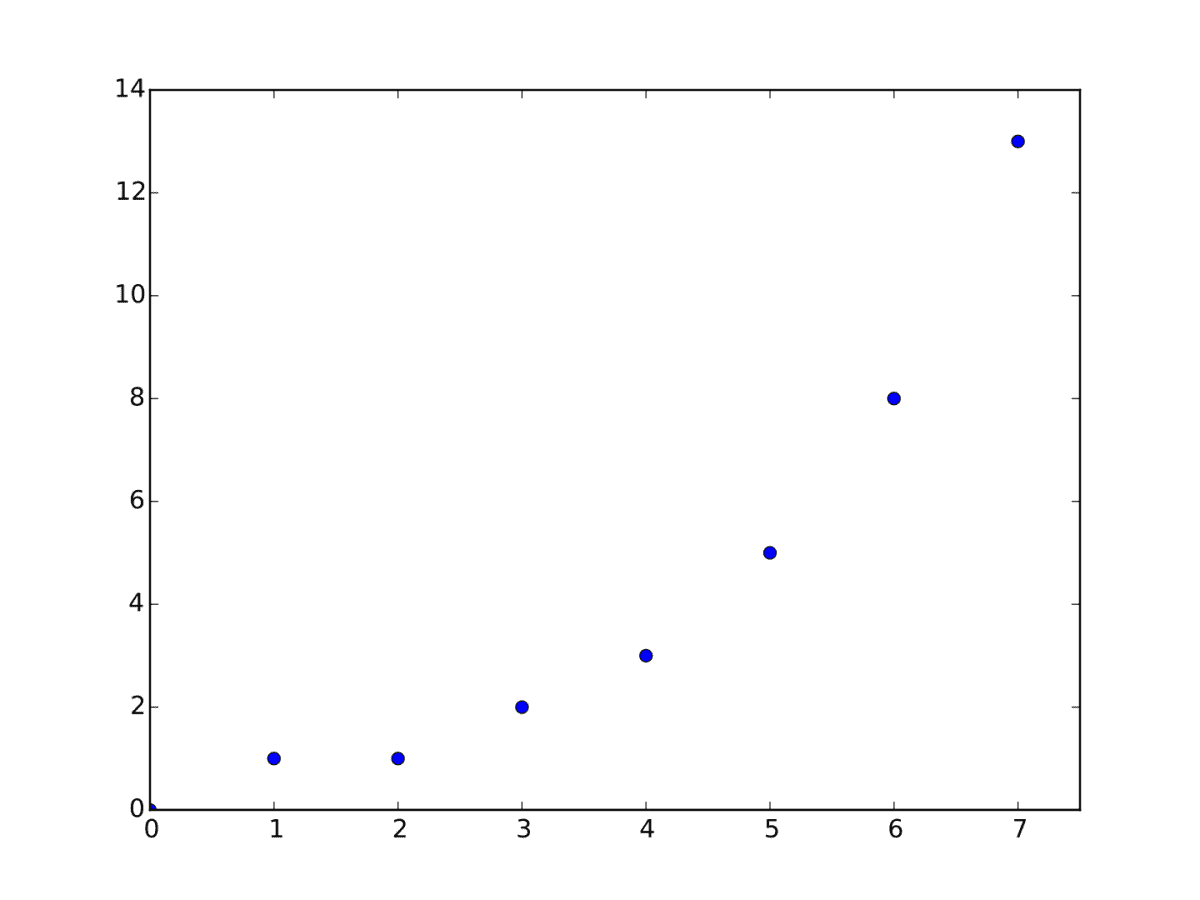The graph of the first several Fibonacci numbers is not linear, which shows us that it is not an arithmetic sequence.

## Conclusion

Now you know what arithmetic sequences are and what they look like.  You also know the answers to some common questions about arithmetic sequences.